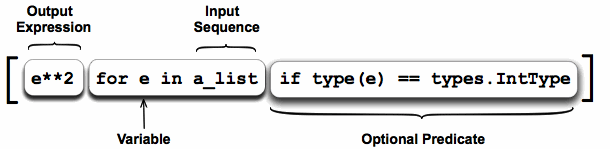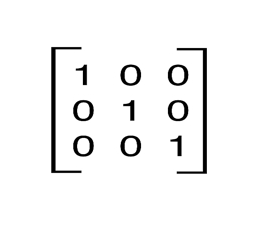# Comprehensions¶

History: where did they come from?

They require a mind shift.

What makes them so compelling (once you ‘get it’)?

Comprehensions are constructs that allow sequences to be built from other sequences. Python 2.0 introduced list comprehensions and Python 3.0 comes with dictionary and set comprehensions.

## List Comprehensions¶

A list comprehension consists of the following parts:

• An Input Sequence.
• A Variable representing members of the input sequence.
• An Optional Predicate expression.
• An Output Expression producing elements of the output list from members of the Input Sequence that satisfy the predicate.

Say we need to obtain a list of all the integers in a sequence and then square them:

```a_list = [1, ‘4’, 9, ‘a’, 0, 4]

squared_ints = [ e**2 for e in a_list if type(e) == types.IntType ]

print squared_ints
# [ 1, 81, 0, 16 ]
```• The iterator part iterates through each member e of the input sequence a_list.
• The predicate checks if the member is an integer.
• If the member is an integer then it is passed to the output expression, squared, to become a member of the output list.

Much the same results can be achieved using the built in functions, map, filter and the anonymous lambda function.

The filter function applies a predicate to a sequence:

```filter(lambda e: type(e) == types.IntType, a_list)
```

Map modifies each member of a sequence:

```map(lambda e: e**2, a_list)
```

The two can be combined:

```map(lambda e: e**2, filter(lambda e: type(e) == types.IntType, a_list))
```

The above example involves function calls to map, filter, type and two calls to lambda. Function calls in Python are expensive. Furthermore the input sequence is traversed through twice and an intermediate list is produced by filter.

The list comprehension is enclosed within a list so, it is immediately evident that a list is being produced. There is only one function call to type and no call to the cryptic lambda instead the list comprehension uses a conventional iterator, an expression and an if expression for the optional predicate.

## Nested Comprehensions¶

An identity matrix of size n is an n by n square matrix with ones on the main diagonal and zeros elsewhere. A 3 by 3 identity matrix is:In python we can represent such a matrix by a list of lists, where each sub-list represents a row. A 3 by 3 matrix would be represented by the following list:

```[ [ 1, 0, 0 ],
[ 0, 1, 0 ],
[ 0, 0, 1 ] ]
```

The above matrix can be generated by the following comprehension:

```[ [ 1 if item_idx == row_idx else 0 for item_idx in range(0, 3) ] for row_idx in range(0, 3) ]
```

## Techniques¶

Using `zip()` and dealing with two or more elements at a time:

```['%s=%s' % (n, v) for n, v in zip(self.all_names, self)]
```

Multiple types (auto unpacking of a tuple):

```[f(v) for (n, f), v in zip(cls.all_slots, values)]
```

A two-level list comprehension using `os.walk()`:

```# Comprehensions/os_walk_comprehension.py
import os
restFiles = [os.path.join(d, f) for d in os.walk(".")
for f in d if f.endswith(".rst")]
for r in restFiles:
print(r)
```

## A More Complex Example¶

Note

This will get a full description of all parts.

```# CodeManager.py
"""
TODO: Break check into two pieces?
TODO: update() is still only in test mode; doesn't actually work yet.

Extracts, displays, checks and updates code examples in restructured text (.rst)
files.

You can just put in the codeMarker and the (indented) first line (containing the
file path) into your restructured text file, then run the update program to
automatically insert the rest of the file.
"""
import os, re, sys, shutil, inspect, difflib

restFiles = [os.path.join(d, f) for d in os.walk(".") if not "_test" in d
for f in d if f.endswith(".rst")]

class Languages:
"Strategy design pattern"

class Python:
codeMarker = "::\n\n"
commentTag = "#"
listings = re.compile("::\n\n( {4}#.*(?:\n+ {4}.*)*)")

class Java:
codeMarker = "..  code-block:: java\n\n"
commentTag = "//"
listings = \
re.compile(".. *code-block:: *java\n\n( {4}//.*(?:\n+ {4}.*)*)")

def shift(listing):
"Shift the listing left by 4 spaces"
return [x[4:] if x.startswith("    ") else x for x in listing.splitlines()]

# TEST - makes duplicates of the rst files in a test directory to test update():
dirs = set([os.path.join("_test", os.path.dirname(f)) for f in restFiles])
if [os.makedirs(d) for d in dirs if not os.path.exists(d)]:
[shutil.copy(f, os.path.join("_test", f)) for f in restFiles]
testFiles = [os.path.join(d, f) for d in os.walk("_test")
for f in d if f.endswith(".rst")]

class Commands:
"""
Each static method can be called from the command line. Add a new static
method here to add a new command to the program.
"""

@staticmethod
def display(language):
"""
Print all the code listings in the .rst files.
"""
for f in restFiles:
if not listings: continue
print('=' * 60 + "\n" + f + "\n" + '=' * 60)
for n, l in enumerate(listings):
print("\n".join(shift(l)))
if n < len(listings) - 1:
print('-' * 60)

@staticmethod
def extract(language):
"""
Pull the code listings from the .rst files and write each listing into
its own file. Will not overwrite if code files and .rst files disagree
unless you say "extract -force".
"""
force = len(sys.argv) == 3 and sys.argv == '-force'
paths = set()
for listing in [shift(listing) for f in restFiles
path = listing[len(language.commentTag):].strip()
if path in paths:
print("ERROR: Duplicate file name: %s" % path)
sys.exit(1)
else:
path = os.path.join("..", "code", path)
dirname = os.path.dirname(path)
if dirname and not os.path.exists(dirname):
os.makedirs(dirname)
if os.path.exists(path) and not force:
if i.startswith("+ ") or i.startswith("- "):
print("ERROR: Existing file different from .rst")
print("Use 'extract -force' to force overwrite")
Commands.check(language)
return
file(path, 'w').write("\n".join(listing))

@staticmethod
def check(language):
"""
Ensure that external code files exist and check which external files
have changed from what's in the .rst files. Generate files in the
_deltas subdirectory showing what has changed.
"""
class Result: # Messenger
def __init__(self, **kwargs):
self.__dict__ = kwargs
result = Result(missing = [], deltas = [])
listings = [Result(code = shift(code), file = f)
for f in restFiles for code in
paths = [os.path.normpath(os.path.join("..", "code", path)) for path in
[listing.code.strip()[len(language.commentTag):].strip()
for listing in listings]]
if os.path.exists("_deltas"):
shutil.rmtree("_deltas")
for path, listing in zip(paths, listings):
if not os.path.exists(path):
result.missing.append(path)
else:
for i in difflib.ndiff(listing.code, code):
if i.startswith("+ ") or i.startswith("- "):
d = difflib.HtmlDiff()
if not os.path.exists("_deltas"):
os.makedirs("_deltas")
html = os.path.join("_deltas",
os.path.basename(path).split('.') + ".html")
open(html, 'w').write(
"<html><h1>Left: %s<br>Right: %s</h1>" %
(listing.file, path) +
d.make_file(listing.code, code))
result.deltas.append(Result(file = listing.file,
path = path, html = html, code = code))
break
if result.missing:
print("Missing %s files:\n%s" %
(language.__name__, "\n".join(result.missing)))
for delta in result.deltas:
print("%s changed in %s; see %s" %
(delta.file, delta.path, delta.html))
return result

@staticmethod
def update(language): # Test until it is trustworthy
"""
Refresh external code files into .rst files.
"""
check_result = Commands.check(language)
if check_result.missing:
print(language.__name__, "update aborted")
return
changed = False
def _update(matchobj):
listing = shift(matchobj.group(1))
path = listing.strip()[len(language.commentTag):].strip()
filename = os.path.basename(path).split('.')
path = os.path.join("..", "code", path)
return language.codeMarker + \
"\n".join([("    " + line).rstrip() for line in listing])
for f in testFiles:
open(f, 'w').write(updated)

if __name__ == "__main__":
commands = dict(inspect.getmembers(Commands, inspect.isfunction))
if len(sys.argv) < 2 or sys.argv not in commands:
print("Command line options:\n")
for name in commands:
print(name + ": " + commands[name].__doc__)
else:
for language in inspect.getmembers(Languages, inspect.isclass):
commands[sys.argv](language)
```

## Set Comprehensions¶

Set comprehensions allow sets to be constructed using the same principles as list comprehensions, the only difference is that resulting sequence is a set.

Say we have a list of names. The list can contain names which only differ in the case used to represent them, duplicates and names consisting of only one character. We are only interested in names longer then one character and wish to represent all names in the same format: The first letter should be capitalised, all other characters should be lower case.

Given the list:

```names = [ 'Bob', 'JOHN', 'alice', 'bob', 'ALICE', 'J', 'Bob' ]
```

We require the set:

```{ 'Bob', 'John', 'Alice' }
```

Note the new syntax for denoting a set. Members are enclosed in curly braces.

The following set comprehension accomplishes this:

```{ name.upper() + name[1:].lower() for name in names if len(name) > 1 }
```

## Dictionary Comprehensions¶

Say we have a dictionary the keys of which are characters and the values of which map to the number of times that character appears in some text. The dictionary currently distinguishes between upper and lower case characters.

We require a dictionary in which the occurrences of upper and lower case characters are combined:

```mcase = {'a':10, 'b': 34, 'A': 7, 'Z':3}

mcase_frequency = { k.lower() : mcase.get(k.lower(), 0) + mcase.get(k.upper(), 0) for k in mcase.keys() }

# mcase_frequency == {'a': 17, 'z': 3, 'b': 34}
```

Note

Contributions by Michael Charlton, 3/23/09